# 3D Theory - OpenGL Rendering

## Rendering Pipeline

Usually when working with openGL object coordinates are represented by a 4 dimensional vector where the 4th number is always 1:

 x y z 1

A general transformation to include both rotation and translation can then be represented using a 4x4 matrix as described on this page:

 r00 r01 r02 t0 r10 r11 r12 t1 r20 r21 r22 t2 0 0 0 1

Where: rij represents the rotation terms and ti represents the translation

However, once the objects coordinates have been calculated, we then need to project the result onto a two dimensional screen. To do this we apply the projection matrix, normailise by dividing all terms by w, the x and y values then represent the position on the screen and the z value is used in z ordering to work out what is in front.

A Projection matrix for a frustum projection is: (see openGL documentation for glFrustum for further information).

 FD/aspect 0 0 0 0 FD 0 0 0 0 (zFar + zNear)/(zFar - zNear) -1 0 0 (2 * zFar * zNear)/(zFar - zNear) 0

So, to summarize, the sequence is as follows:

 Model-View matrix [x,y,z,1] Projection matrix [x,y,z,w] Perspective division [x/w,y/w,z/w,1] Viewport Transformation [u,v]

This is all based on projective geometry which is explained on this page.

### Object coordinatesPoints in 3D space are represented by 3 numbers represented by its position in the x, y and z planes.

### Normalized coordinates - frustum projection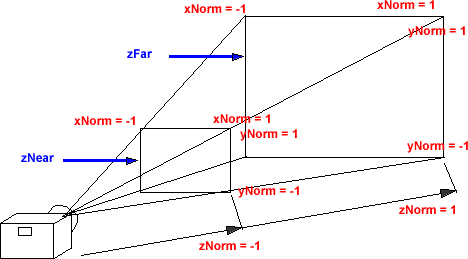In order to represent this on a 2D screen we need to define a projection. A frustum projection shows an object, which is closer to the camera as larger than an object which is further away. To do this, the x and y coordinates are scaled by an amount inversely proportional to the position in the z dimension.

### Screen coordinates - frustum projection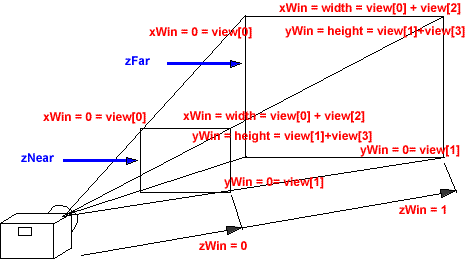The viewport transformation scales and shifts the normalised coordinates to fit the size of the screen.

### Pipeline

The rendering pipeline takes the model data and renders it on the screen. This is done partly by software and partly by hardware, the boundary between these depends on what graphics card and operating system that you are using.

As shown in the diagrams above, in a frustum projection, the x and y coordinates are scaled by an amount inversely proportional to the position in the z dimension. A 3x3 matrix cannot represent such a transformation, so in the pipeline an additional variable 'w' is added to each coordinate. This is a scaling value, used to scale the x,y and z values depending on the inverse of its distance from the camera.

To do this, the matrices are increased to 4x4 to include this scaling value.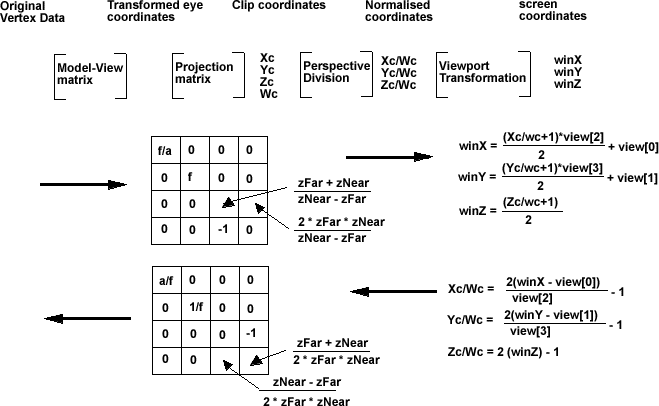### Projection Matrix

fd=1/Math::Tan(fovY/2);
aspect = ((double)Width/(double)(Height-30));

example (width=258 height=440) Aspect=0.629268
FD/aspect=5.93077841512884
FD=3.73205080756888

projection->m00 = FD/aspect;
projection->m01 = 0;
projection->m02 = 0;
projection->m03 = 0;
projection->m10 = 0;
projection->m11 = FD;
projection->m12 = 0;
projection->m13 = 0;
projection->m20 = 0;
projection->m21 = 0;
projection->m22 = (zFar + zNear)/(zFar - zNear);
projection->m23 = -1;
projection->m30 = 0;
projection->m31 = 0;
projection->m32 = (2 * zFar * zNear)/(zFar - zNear);
projection->m33 = 0;

### Inverse Projection Matrix

mi00 = - FD*m23*m32 / det = aspect/FD

in example above = 0.16861193084690149207499690607832
mi01 = 0
mi02 = 0
mi03 = 0
mi10 = 0
mi11 = - FD/aspect*m23*m32 /det = 1/FD

in example above = 0.26794919243112251215656695782073
mi12 = 0
mi13 = 0
mi20 = 0
mi21 = 0
mi22 = 0
mi23 = - FD/aspect*FD*m23/det = 1/m32 = (zNear-zFar)/(2 * zFar * zNear)

assuming zFar = -20 and zNear=-3 then m23 = 17/(2 * 20 * 3) =0.141666667
mi30 = 0
mi31 = 0
mi32 = m02*m11*m30 - m01*m12*m30 - m02*m10*m31 + m00*m12*m31 + m01*m10*m32 - m00*m11*m32;
= - m00*m11*m32;
= - FD/aspect*FD*m32/det
= 1/m23
= -1
mi33 = + FD/aspect*FD*m22/det = -m22/(m23 * m32) = m22/m32 = (zFar+zNear) / (2*zFar*zNear)

assuming zFar = -20 and zNear=-3 then m33 = -23 / (2*20*3) = -23/120 = 0.19166667

det= m03 * m12 * m21 * m30-m02 * m13 * m21 * m30-m03 * m11 * m22 * m30+m01 * m13 * m22 * m30+
m02 * m11 * m23 * m30-m01 * m12 * m23 * m30-m03 * m12 * m20 * m31+m02 * m13 * m20 * m31+
m03 * m10 * m22 * m31-m00 * m13 * m22 * m31-m02 * m10 * m23 * m31+m00 * m12 * m23 * m31+
m03 * m11 * m20 * m32-m01 * m13 * m20 * m32-m03 * m10 * m21 * m32+m00 * m13 * m21 * m32+
m01 * m10 * m23 * m32-m00 * m11 * m23 * m32-m02 * m11 * m20 * m33+m01 * m12 * m20 * m33+
m02 * m10 * m21 * m33-m00 * m12 * m21 * m33-m01 * m10 * m22 * m33+m00 * m11 * m22 * m33;
= -m00 * m11 * m23 * m32;
=FD/aspect * FD * m23 * m32

[0.168611930846902 0 0 0]
[0 0.267949192431123 0 0]
[0 0 0 0.141666666666667]
[0 0 -1 0.191666666666667]

## Picking

How to do reverse process, select 3d object from 2d position on screen,1. generate the ray
2. traverse the scenegraph to pick the nearest object along the scenegraph

how to pick the nearest object

metadata block
see also:

Correspondence about this page

Book Shop - Further reading.

Where I can, I have put links to Amazon for books that are relevant to the subject, click on the appropriate country flag to get more details of the book or to buy it from them.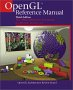If you are doing a lot of OpenGL programming, and you can afford it. It really helps (in fact its almost essential) to have these 2 books at hand. The programming guide (red cover) explains the theory and the reference manual (blue cover) has complete definitions for all the API calls.

Commercial Software Shop

Where I can, I have put links to Amazon for commercial software, not directly related to this site, but related to the subject being discussed, click on the appropriate country flag to get more details of the software or to buy it from them.Dark Basic Professional Edition - It is better to get this professional edition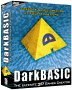This is a version of basic designed for building games, for example to rotate a cube you might do the following: make object cube 1,100 for x=1 to 360 rotate object 1,x,x,0 next x

This site may have errors. Don't use for critical systems.

Copyright (c) 1998-2020 Martin John Baker - All rights reserved - privacy policy.